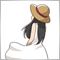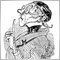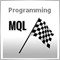# Indicator window blank89

Trend Direction Force Indicator made by mladen, it compiles without any errors and should work but indicator window is blank. Tried on 3 different broker's MT4 but nothing.

```//+------------------------------------------------------------------+
//|                                Trend direction & force index.mq4 |
//|                                                                  |
//|                                                                  |
//| original metastock indicator made by Piotr Wojdylo               |
//+------------------------------------------------------------------+

#property indicator_separate_window
#property indicator_buffers  7
#property indicator_color1   DimGray
#property indicator_color2   DimGray
#property indicator_color3   DimGray
#property indicator_color4   DeepSkyBlue
#property indicator_color5   DeepSkyBlue
#property indicator_color6   PaleVioletRed
#property indicator_color7   PaleVioletRed
#property indicator_width3   2
#property indicator_width4   2
#property indicator_width5   2
#property indicator_width6   2
#property indicator_width7   2
#property indicator_maximum  1
#property indicator_minimum -1

//
//
//
//
//

extern string TimeFrame              = "Current time frame";
extern int    trendPeriod            = 20;
extern double TriggerUp              =  0.05;
extern double TriggerDown            = -0.05;
extern double SmoothLength           = 5;
extern double SmoothPhase            = 0;
extern bool   ColorChangeOnZeroCross = false;
extern bool   Interpolate            = true;

//
//
//
//
//

double   TrendBuffer[];
double   TrendBufferUa[];
double   TrendBufferUb[];
double   TrendBufferDa[];
double   TrendBufferDb[];
double   TriggBuffera[];
double   TriggBufferb[];
double   trend[];

//
//
//
//
//

string indicatorFileName;
bool   calculateValue;
bool   returnBars;
int    timeFrame;

//+------------------------------------------------------------------+
//|                                                                  |
//+------------------------------------------------------------------+
//
//
//
//
//

int init()
{
IndicatorBuffers(8);
SetIndexBuffer(0,TriggBuffera);  SetIndexLabel(0,NULL);
SetIndexBuffer(1,TriggBufferb);  SetIndexLabel(1,NULL);
SetIndexBuffer(2,TrendBuffer);   SetIndexLabel(2,"Trend direction & force");
SetIndexBuffer(3,TrendBufferUa);
SetIndexBuffer(4,TrendBufferUb);
SetIndexBuffer(5,TrendBufferDa);
SetIndexBuffer(6,TrendBufferDb);
SetIndexBuffer(7,trend);

//
//
//
//
//

indicatorFileName = WindowExpertName();
calculateValue    = (TimeFrame=="CalculateValue"); if (calculateValue) return(0);
returnBars        = (TimeFrame=="returnBars");     if (returnBars)     return(0);
timeFrame         = stringToTimeFrame(TimeFrame);

//
//
//
//
//

IndicatorShortName(timeFrameToString(timeFrame)+" trend direction & force ("+trendPeriod+")");
return(0);
}
int deinit() { return(0); }

//+------------------------------------------------------------------+
//|                                                                  |
//+------------------------------------------------------------------+
//
//
//
//
//

double workTrend[];
#define _MMA   0
#define _SMMA  1
#define _TDF   2

//
//
//
//
//

int start()
{
int i,r,limit,counted_bars=IndicatorCounted();

if(counted_bars < 0) return(-1);
if(counted_bars>0) counted_bars--;
limit = MathMin(Bars-counted_bars,Bars-1);
if (returnBars) { TriggBuffera = limit+1; return(0); }

//
//
//
//
//

if (calculateValue || timeFrame == Period())
{
if (ArrayRange(workTrend,0)!=Bars) ArrayResize(workTrend,Bars);
if (trend[limit]== 1) CleanPoint(limit,TrendBufferUa,TrendBufferUb);
if (trend[limit]==-1) CleanPoint(limit,TrendBufferDa,TrendBufferDb);

//
//
//
//
//

double alpha = 2.0 /(trendPeriod+1.0);
for (i=limit, r=Bars-i-1; i>=0; i--, r++)
{
workTrend[r][_MMA]  = iMA(NULL,0,trendPeriod,0,MODE_EMA,PRICE_CLOSE,i);
workTrend[r][_SMMA] = workTrend[r-1][_SMMA]+alpha*(workTrend[r][_MMA]-workTrend[r-1][_SMMA]);
double impetmma  = workTrend[r][_MMA]  - workTrend[r-1][_MMA];
double impetsmma = workTrend[r][_SMMA] - workTrend[r-1][_SMMA];
double divma     = MathAbs(workTrend[r][_MMA]-workTrend[r][_SMMA])/Point;
double averimpet = (impetmma+impetsmma)/(2*Point);
workTrend[r][_TDF]  = divma*MathPow(averimpet,3);

//
//
//
//
//

double absValue = absHighest(workTrend,_TDF,trendPeriod*3,r);
if (absValue > 0)
TrendBuffer[i]  = iSmooth(workTrend[r][_TDF]/absValue,SmoothLength,SmoothPhase,i);
else  TrendBuffer[i]  = iSmooth(                       0.00,SmoothLength,SmoothPhase,i);
TriggBuffera[i] = TriggerUp;
TriggBufferb[i] = TriggerDown;

//
//
//
//
//

TrendBufferUa[i] = EMPTY_VALUE;
TrendBufferUb[i] = EMPTY_VALUE;
TrendBufferDa[i] = EMPTY_VALUE;
TrendBufferDb[i] = EMPTY_VALUE;
trend[i]         = trend[i+1];
if (ColorChangeOnZeroCross)
{
if (TrendBuffer[i]>0) trend[i] =  1;
if (TrendBuffer[i]<0) trend[i] = -1;
}
else
{
if (TrendBuffer[i]>TriggBuffera[i])                                   trend[i] =  1;
if (TrendBuffer[i]<TriggBufferb[i])                                   trend[i] = -1;
if (TrendBuffer[i]>TriggBufferb[i] && TrendBuffer[i]<TriggBuffera[i]) trend[i] =  0;
}
if (trend[i] ==  1) PlotPoint(i,TrendBufferUa,TrendBufferUb,TrendBuffer);
if (trend[i] == -1) PlotPoint(i,TrendBufferDa,TrendBufferDb,TrendBuffer);
}
return(0);
}

//
//
//
//
//

limit = MathMax(limit,MathMin(Bars,iCustom(NULL,timeFrame,indicatorFileName,"returnBars",0)*timeFrame/Period()));
for(i=limit; i>=0; i--)
{
int y = iBarShift(NULL,timeFrame,Time[i]);
TrendBufferUa[i] = EMPTY_VALUE;
TrendBufferUb[i] = EMPTY_VALUE;
TrendBufferDa[i] = EMPTY_VALUE;
TrendBufferDb[i] = EMPTY_VALUE;
TriggBuffera[i]  = TriggerUp;
TriggBufferb[i]  = TriggerDown;

//
//
//
//
//

if (!Interpolate || y==iBarShift(NULL,timeFrame,Time[i-1])) continue;

//
//
//
//
//

datetime time = iTime(NULL,timeFrame,y);
for(int n = 1; i+n < Bars && Time[i+n] >= time; n++) continue;
for(int k = 1; k < n; k++)
TrendBuffer[i+k] = TrendBuffer[i] + (TrendBuffer[i+n]-TrendBuffer[i])*k/n;
}
for(i=limit; i>=0; i--)
{
if (trend[i] ==  1) PlotPoint(i,TrendBufferUa,TrendBufferUb,TrendBuffer);
if (trend[i] == -1) PlotPoint(i,TrendBufferDa,TrendBufferDb,TrendBuffer);
}

//
//
//
//
//

return(0);

}

//------------------------------------------------------------------
//
//------------------------------------------------------------------
//
//
//
//
//

{
{
int whichBar = 0;
else     whichBar = 1;

//
//
//
//
//

Comment(trend[whichBar],"   ",trend[whichBar+1]);
if (ColorChangeOnZeroCross)
{
if (trend[whichBar] != trend[whichBar+1])
{
if (trend[whichBar] == 1) doAlert(whichBar," crossed zero line up");
if (trend[whichBar] ==-1) doAlert(whichBar," crossed zero line down");
}
}
else
{
if (trend[whichBar] != trend[whichBar+1])
{
if (trend[whichBar]   == 1                        ) doAlert(whichBar," crossed "+DoubleToStr(TriggerUp  ,2)+" line up");
if (trend[whichBar]   ==-1                        ) doAlert(whichBar," crossed "+DoubleToStr(TriggerDown,2)+" line down");
if (trend[whichBar+1] == 1 && trend[whichBar] == 0) doAlert(whichBar," crossed "+DoubleToStr(TriggerUp  ,2)+" line down");
if (trend[whichBar+1] ==-1 && trend[whichBar] ==-0) doAlert(whichBar," crossed "+DoubleToStr(TriggerDown,2)+" line up");
}
}
}
}

//
//
//
//
//

{
static datetime previousTime;
string message;

if (previousAlert != doWhat || previousTime != Time[forBar]) {
previousTime   = Time[forBar];

//
//
//
//
//

message =  timeFrameToString(Period())+" "+Symbol()+" at "+TimeToStr(TimeLocal(),TIME_SECONDS)+doWhat;
if (alertsEmail)   SendMail(StringConcatenate(Symbol()," trend dirrection & strength "),message);
}
}

//+------------------------------------------------------------------+
//|                                                                  |
//+------------------------------------------------------------------+

double absHighest(double& array[][], int index, int length, int shift)
{
double result = 0.00;

for (int i = length-1; i>=0; i--)
if (result < MathAbs(array[shift-i][index]))
result = MathAbs(array[shift-i][index]);
return(result);
}

//+------------------------------------------------------------------+
//|                                                                  |
//+------------------------------------------------------------------+
//
//
//
//
//

void CleanPoint(int i,double& first[],double& second[])
{
if ((second[i]  != EMPTY_VALUE) && (second[i+1] != EMPTY_VALUE))
second[i+1] = EMPTY_VALUE;
else
if ((first[i] != EMPTY_VALUE) && (first[i+1] != EMPTY_VALUE) && (first[i+2] == EMPTY_VALUE))
first[i+1] = EMPTY_VALUE;
}

//
//
//
//
//

void PlotPoint(int i,double& first[],double& second[],double& from[])
{
if (first[i+1] == EMPTY_VALUE)
{
if (first[i+2] == EMPTY_VALUE) {
first[i]   = from[i];
first[i+1] = from[i+1];
second[i]  = EMPTY_VALUE;
}
else {
second[i]   =  from[i];
second[i+1] =  from[i+1];
first[i]    = EMPTY_VALUE;
}
}
else
{
first[i]  = from[i];
second[i] = EMPTY_VALUE;
}
}

//+-------------------------------------------------------------------
//|
//+-------------------------------------------------------------------
//
//
//
//
//

string sTfTable[] = {"M1","M5","M15","M30","H1","H4","D1","W1","MN"};
int    iTfTable[] = {1,5,15,30,60,240,1440,10080,43200};

//
//
//
//
//

int stringToTimeFrame(string tfs)
{
tfs = stringUpperCase(tfs);
for (int i=ArraySize(iTfTable)-1; i>=0; i--)
if (tfs==sTfTable[i] || tfs==""+iTfTable[i]) return(MathMax(iTfTable[i],Period()));
return(Period());
}
string timeFrameToString(int tf)
{
for (int i=ArraySize(iTfTable)-1; i>=0; i--)
if (tf==iTfTable[i]) return(sTfTable[i]);
return("");
}

//
//
//
//
//

string stringUpperCase(string str)
{
string   s = str;

for (int length=StringLen(str)-1; length>=0; length--)
{
int tchar = StringGetChar(s, length);
if((tchar > 96 && tchar < 123) || (tchar > 223 && tchar < 256))
s = StringSetChar(s, length, tchar - 32);
else if(tchar > -33 && tchar < 0)
s = StringSetChar(s, length, tchar + 224);
}
return(s);
}

//+------------------------------------------------------------------+
//|                                                                  |
//+------------------------------------------------------------------+
//
//
//
//
//

double wrk[];

#define bsmax  5
#define bsmin  6
#define volty  7
#define vsum   8
#define avolty 9

//
//
//
//
//

double iSmooth(double price, double length, double phase, int i, int s=0)
{
if (length <=1) return(price);
if (ArrayRange(wrk,0) != Bars) ArrayResize(wrk,Bars);

int r = Bars-i-1;
if (r==0) { for(int k=0; k<7; k++) wrk[r][k+s]=price; for(; k<10; k++) wrk[r][k+s]=0; return(price); }

//
//
//
//
//

double len1   = MathMax(MathLog(MathSqrt(0.5*(length-1)))/MathLog(2.0)+2.0,0);
double pow1   = MathMax(len1-2.0,0.5);
double del1   = price - wrk[r-1][bsmax+s];
double del2   = price - wrk[r-1][bsmin+s];
double div    = 1.0/(10.0+10.0*(MathMin(MathMax(length-10,0),100))/100);
int    forBar = MathMin(r,10);

wrk[r][volty+s] = 0;
if(MathAbs(del1) > MathAbs(del2)) wrk[r][volty+s] = MathAbs(del1);
if(MathAbs(del1) < MathAbs(del2)) wrk[r][volty+s] = MathAbs(del2);
wrk[r][vsum+s] =       wrk[r-1][vsum+s] + (wrk[r][volty+s]-wrk[r-forBar][volty+s])*div;

//
//
//
//
//

wrk[r][avolty+s] = wrk[r-1][avolty+s]+(2.0/(MathMax(4.0*length,30)+1.0))*(wrk[r][vsum+s]-wrk[r-1][avolty+s]);
if (wrk[r][avolty+s] > 0)
double dVolty = wrk[r][volty+s]/wrk[r][avolty+s]; else dVolty = 0;
if (dVolty > MathPow(len1,1.0/pow1)) dVolty = MathPow(len1,1.0/pow1);
if (dVolty < 1)                      dVolty = 1.0;

//
//
//
//
//

double pow2 = MathPow(dVolty, pow1);
double len2 = MathSqrt(0.5*(length-1))*len1;
double Kv   = MathPow(len2/(len2+1), MathSqrt(pow2));

if (del1 > 0) wrk[r][bsmax+s] = price; else wrk[r][bsmax+s] = price - Kv*del1;
if (del2 < 0) wrk[r][bsmin+s] = price; else wrk[r][bsmin+s] = price - Kv*del2;

//
//
//
//
//

double R     = MathMax(MathMin(phase,100),-100)/100.0 + 1.5;
double beta  = 0.45*(length-1)/(0.45*(length-1)+2);
double alpha = MathPow(beta,pow2);

wrk[r][0+s] = price + alpha*(wrk[r-1][0+s]-price);
wrk[r][1+s] = (price - wrk[r][0+s])*(1-beta) + beta*wrk[r-1][1+s];
wrk[r][2+s] = (wrk[r][0+s] + R*wrk[r][1+s]);
wrk[r][3+s] = (wrk[r][2+s] - wrk[r-1][4+s])*MathPow((1-alpha),2) + MathPow(alpha,2)*wrk[r-1][3+s];
wrk[r][4+s] = (wrk[r-1][4+s] + wrk[r][3+s]);

//
//
//
//
//

return(wrk[r][4+s]);
}```504

laurixx:

Trend Direction Force Indicator made by mladen, it compiles without any errors and should work but indicator window is blank. Tried on 3 different broker's MT4 but nothing.

Forex Station
• www.forex-station.com
Since it is repeating itself over and over, here is a description and a "fix" for one of the nasty bugs of build 1090 of mt4Moderator
8632

I think(!) that one need not to change something, but just do (try!):

1. "Save under different name"
2. Select the same name!
3. Upon the remark: .."file exists" press yes (or ok?)
4. Compile it again.
5. Attach it to the chart - and see whether or not it worked!

Once and a while the debug-button is greyed (=not startable) and this procedure enables the debugger again.89

Brilliant! Thank you!32790

laurixx:

Trend Direction Force Indicator made by mladen, it compiles without any errors and should work but indicator window is blank. Tried on 3 different broker's MT4 but nothing.

In fact, the problem is that there are no styles for drawing lines.

Simply insert the drawing styles into init() and the problem will be gone.

```   SetIndexStyle(0,DRAW_LINE);
SetIndexStyle(1,DRAW_LINE);
SetIndexStyle(2,DRAW_LINE);
SetIndexStyle(3,DRAW_LINE);
SetIndexStyle(4,DRAW_LINE);
SetIndexStyle(5,DRAW_LINE);
SetIndexStyle(6,DRAW_LINE);```19988

Alexander Voronkov: Simply insert the drawing styles into init() and the problem will be gone.

That isn't the problem; the problem is compiling a working indicator sometimes results in no drawing but the data window has values. Compiling a second or a third time fixes it with no changes to the code.154224

Alexander Voronkov:

In fact, the problem is that there are no styles for drawing lines.

Simply insert the drawing styles into init() and the problem will be gone.

The way you wrote it, it is not a style. That is drawing type. And it is obvious that mt4 now sometimes "forgets" what used to be default drawing type (line) for years and years (but does not "forget" it always - "just" sometimes :)).

In any case the issue is clear : it sometimes displays lines and sometimes it does not (even though they are visible in the data window). Ie: that is a bug 101% (see post too) since without any change of code whatsoever it will sometimes be displayed and sometimes not. If it did not show it all the time then it would be a change in mt4. Since it sometimes draws those lines and sometimes it does not it is a bug by all the definitions of the bug there are32790

The way you wrote it, it is not a style. That is drawing type. And it is obvious that mt4 now sometimes "forgets" what used to be default drawing type (line) for years and years (but does not "forget" it always - "just" sometimes :)).

In any case the issue is clear : it sometimes displays lines and sometimes it does not (even though they are visible in the data window). Ie: that is a bug 101% (see post too) since without any change of code whatsoever it will sometimes be displayed and sometimes not. If it did not show it all the time then it would be a change in mt4. Since it sometimes draws those lines and sometimes it does not it is a bug by all the definitions of the bug there are

Type or style, it does not really matter and the problem is connected with this, not paying attention to someone's messages and someone's mistakes!32790

whroeder1:

That isn't the problem; the problem is compiling a working indicator sometimes results in no drawing but the data window has values. Compiling a second or a third time fixes it with no changes to the code.

If this were not a problem, then no one would have addressed such requests!154224

Alexander Voronkov:

Type or style, it does not really matter and the problem is connected with this, not paying attention to someone's messages and someone's mistakes!

Please then explain why it sometimes produces correct ex4 file (that works) without any code change and sometimes incorrect ex4 file (that does not work) when exactly the same code is repeatedly compiled

Patch is a patch (and nobody denies that there are patches that can fix that bug/issue - use #property indicator_type(nnn)  DRAW_LINE for example and no need to do anything in the init). All that "issue" started with the build 1090 and all that code was perfectly "legal" till this unfortunate build 1090 was released. Hence "patches" for builds pre build 1090 were not necessary since mt4 was working as it used to work for years and years till this build 1090. All that code was working perfectly well (as it was supposed to work, and not just that indicator but 1000s of indicators) - why should it have been changed then in any way when there were no "mistakes" in it?

But bug is a bug also - and in this case it is a MT4 bug. Closing eyes on that will not remove it

Now it is up to MQ: are they going to force this as a new requirement (and make something similar as with build 600) just because default drawing type that was valid for all the code before build 1090 is not valid any more (but funny enough, just sometimes, since it sometimes works) and that way make one more time people forcefully change code that worked for years and years back (and that code from post one was written years and years ago - long before build 1090) or they are going to fix the bug so that it stops producing correct ex4 files - randomly?

All the best32790

Please then explain why it sometimes produces correct ex4 file (that works) without any code change and sometimes incorrect ex4 file (that does not work) when exactly the same code is repeatedly compiled

Patch is a patch (and nobody denies that there are patches that can fix that bug/issue - use #property indicator_type(nnn)  DRAW_LINE for example and no need to do anything in the init). All that "issue" started with the build 1090 and all that code was perfectly "legal" till this unfortunate build 1090 was released. Hence "patches" for builds pre build 1090 were not necessary since mt4 was working as it used to work for years and years till this build 1090. All that code was working perfectly well (as it was supposed to work, and not just that indicator but 1000s of indicators) - why should it have been changed then in any way when there were no "mistakes" in it?

But bug is a bug also - and in this case it is a MT4 bug. Closing eyes on that will not remove it

Now it is up to MQ: are they going to force this as a new requirement (and make something similar as with build 600) just because default drawing type that was valid for all the code before build 1090 is not valid any more (but funny enough, just sometimes, since it sometimes works) and that way make one more time people forcefully change code that worked for years and years back (and that code from post one was written years and years ago - long before build 1090) or they are going to fix the bug so that it stops producing correct ex4 files - randomly?

All the best

Mladen, do not worry you so, it certainly is not your fault and I was not going to accuse you of this!

Thank you very much, for the huge number of indicators that you have made, from me and I think from all users who have ever used your codes.

The fact is that this is not the first time I have encountered this problem and get rid of it, in this way.

Whose error is this, this is another question and it will most likely not be solved any more, since MQL4 is no longer developing, and somehow it is necessary to get rid of it, so I suggested this option. You can call ServiceDesk with this problem and ask them to solve this issue.

Regards.